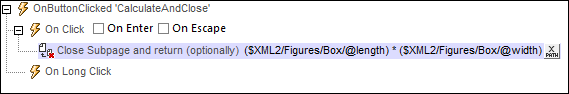# Altova MobileTogether Designer

 Scroll Home Prev Top Next More

The Let action (screenshot below) defines a variable with a value that is set via: (i) an XPath expression, (ii) an Action Group Result, or (iii) a Subpage Result.

To enter the name of the variable, double-click to the right of the \$ sign and type in the variable's name (circled in green in screenshot below).

Select whether you wish to set the value of the variable via an XPath expression, an Action Group Result, or a Subpage Result.

Define one or more child actions of the Let action. For example, the Let action shown in the screenshot below contains an Update Node(s) action, which updates a node with the value of the variable defined in the Let action.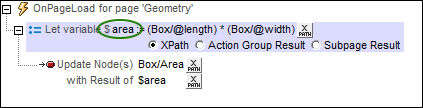Note: The variable defined in a Let action is in scope only within that Let action. This means that it can be used only in child actions of the Let action.

 Note: If a variable contains a nodeset and the nodeset is modified during processing, then the variable is invalidated and cannot be used subsequently.

The XPath option

The XPath option enables you to enter a static value or to generate a dynamic value using XML tree nodes. For example, in the screenshot below, the values of two nodes are multiplied (circled in red). The resulting value will be the value of the variable (\$area). The variable has then been used to update the content of an XML tree node.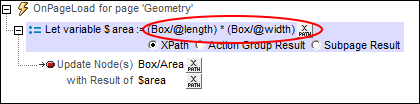The Action Group Result option

The Action Group Result option (screenshot below) sets the value of the variable to be the result of an Action Group. In the screenshot below, we have given the variable a name of \$area, and set its value to be the result of the Action Group called RectangleArea (circled in red below). (All existing Action Groups are available for selection in the variable's combo box.) To edit the Action Group, click the Edit button (circled in green). We have also defined the values of two parameters to be dynamic; they will take their values from XML tree nodes.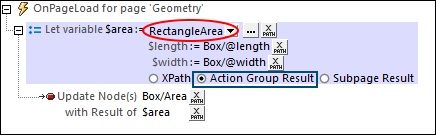The Action Group returns a result via the Return action (see screenshot below). In the screenshot below, for example, we declare two parameters (\$length and \$width); in the Return action, we multiply the values of the two parameters. Note that the values of the parameters are obtained at runtime from the XML tree nodes that have been defined, in the Let action (see screenshot above), as the values of the Let action's parameters.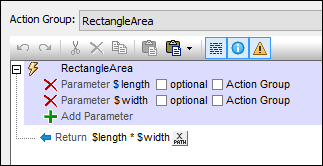The Subpage Result option

The Subpage Result option (screenshot below) sets the value of the variable to be the result of a subpage. This allows a calculation to be carried out on another page. When the subpage is closed, a result can be optionally returned. This result is the Subpage Result that will be used as the value of the variable defined in the Let action. For example, in the screenshot below, the Let action defines a variable called \$area, and sets its value to be the result of the subpage called RectangleArea (circled in red below).

The properties of the Subpage Result option are the same as the Go to Subpage action, and are described in detail there.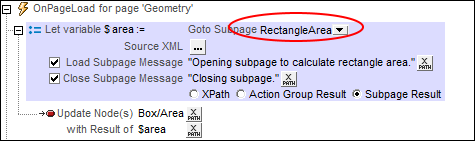At runtime, when the Let action is executed, the subpage is opened and it will be processed as defined in its design. The subpage will be closed when the Close Subpage action is executed. This action has an optional return value which is calculated by an XPath expression (see screenshot below). This returned value will be passed to the Let action and will become the value of the variable defined in the Let action.